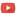KE8QZC | SFW | TSWSyllabus: [pdf]

Previous course materials
Fall 2017 (Fairmont)
Spring 2015 (Missouri S&T)
Fall 2014 (Missouri S&T)
Exams
Exam 1: [pdf] [tex]
Exam 2: [pdf] [tex]
Exam 3: [pdf] [tex]

Homework
Homework 1 (due 26 August) (solution: [pdf]): Section 1.1: #11, 12, 13, 15, 16, 17, 18, 19, 20; Section 2.1: 1, 4, 5, 6, 11, 13, 14, 25, 27, 28, 29, 30, 31, 32; Section 2.2 (note: on problems 9-11, (only do part b)): 1, 2, 3, 4, 9, 10, 11, 25, 26, 27, 28, 32 and the following problem
Problem A: Solve the system of equations, where $i$ denotes the "imaginary number" obeying the property $i^2=-1$: $$\left\{ \begin{array}{llll} iz_1 & + 2iz_2 &= 1 \\ - z_1 &+ iz_2 &= 0 \\ \end{array} \right.$$ note: it is helpful to remember that to "simplify" a complex number of the form $\dfrac{a+bi}{c+di}$ you may multiply by a "convenient form of $1$" defined from the "complex conjugate" of the denominator as follows: $$\dfrac{a+bi}{c+di} = \dfrac{a+bi}{c+di} \dfrac{c-di}{c-di} = \dfrac{(a+bi)(c-di)}{c^2+d^2} = \dfrac{ac + bd}{c^2+d^2} + \dfrac{bc-ad}{c^2+d^2}i.$$

Homework 2 (due 4 September) (solution: [pdf]): Section 2.2 (note: on problems 9-11, only do part b): #1, 2, 3, 4, 9, 10, 11, 25, 26, 27, 28, 32; Section 2.3: 1, 2, 3, 4, 7, 8, 9, 10, 22, 23, 24, 25 and the following problem:
Problem A: Solve the system of equations, where $i$ denotes the "imaginary number" obeying the property $i^2=-1$: $$\left\{ \begin{array}{llll} iz_1 & + 2iz_2 &= 1 \\ - z_1 &+ iz_2 &= 0 \\ \end{array} \right.$$ note: it is helpful to remember that to "simplify" a complex number of the form $\dfrac{a+bi}{c+di}$ you may multiply by a "convenient form of $1$" defined from the "complex conjugate" of the denominator as follows: $$\dfrac{a+bi}{c+di} = \dfrac{a+bi}{c+di} \dfrac{c-di}{c-di} = \dfrac{(a+bi)(c-di)}{c^2+d^2} = \dfrac{ac + bd}{c^2+d^2} + \dfrac{bc-ad}{c^2+d^2}i.$$
Homework 3 (due 9 September) (solution: [pdf]): Section 2.3: 9, 10, 22, 23, 24, 25, 29, 30; Section 3.1: #1, 2, 3, 4, 9, 10, 11, 12, 17, 21, 22, 39, 40
Homework 4 (due 16 September) (solution: [pdf]): Section 3.1: #1, 2, 3, 4, 9, 10, 11, 12, 17, 21, 22, 39; Section 3.2: #2, 4, 5, 7, 14, 15, 37; Section 3.3: #1, 2, 3, 4, 50, 52, 53
Homework 5 (due 2 October) (solution: [pdf]): Section 6.1 #24, 26, 27, 28, 34, 36, 46, 53, 62; Section 6.2: #5, 6, 8, 10, 11, 18, 19, 24, 25, 27, 28, 29, 35, 36, 39
Homework 6 (due 7 October) (solution: [pdf]): Section 6.2: #5, 6, 8, 10, 11, 18, 19, 24, 25, 27, 28, 29, 35, 36, 39; Section 6.3: #2, 3, 7, 8; Section 6.4: #1, 2, 3, 5, 6, 9, 10, 15, 16, 24, 27
Homework 7 (due 14 October) (solution: [pdf]): Section 6.3: #2, 3, 7, 8; Section 6.4: #1, 2, 3, 5, 6, 9, 10, 15, 16, 24, 27
Homework 8 (due 23 October) (solution: [pdf]): Section 6.4: #15, 16, 18, 27, 28
Homework 9 (due 28 October) (solution: [pdf]: Section 6.5: #1, 2, 3, 4, 9, 10, 11, 13, 15, 16, 17, 18, 21, 24, 26, 27; Section 6.6: #1, 2, 3, 4, 5, and the following problem:
Problem A: ("Chebyshev polynomials") Let $T_n\colon \mathscr{P}_2 \rightarrow \mathscr{P}_2$ be defined by $T_n(p)=(1-x^2)p''-xp'+n^2p$ for $n=0,1,2$. Find $\mathrm{ker}(T_n)$ for $n=0,1,2$.
Homework 10 (due 6 November) (solution: [pdf]): Section 4.1: #1, 6, 9, 10, 11, 27, 28; Section 4.2: #1, 3, 6, 8, 10, 47, 48, 49; Section 4.3: #2, 3, 4
Homework 11 (due 15 November) (solution: [pdf]): Section 4.4: #8, 9; recall that if the eigenvalues $\lambda_1, \ldots, \lambda_n$ of the matrix $A$ are all distinct with associated eigenvectors $\vec{v}_1, \ldots, \vec{v}_n$, then the "general solution" of the system of differential equations $\vec{x}'=A\vec{x}$ is $\vec{x}(t)=c_1 e^{\lambda_1 t}\vec{v}_1 + \ldots + c_n e^{\lambda_n t} \vec{v}_n$ -- using this fact, solve the following two systems of differential equations:
(a) $\vec{x}'=\begin{bmatrix} 1&3 \\ 2&0 \end{bmatrix}\vec{x}$
(b) $\vec{x}'=\begin{bmatrix} 1 & -1 & 0 \\ -1 & 2 & -1 \\ 0 & -1 & 1 \end{bmatrix} \vec{x}$.
Homework 12 (due 18 November) (solution: [pdf]): (the "Examples" being referred to appear in the notes here)
Problem A. Let $H=(\mathbb{R}^3,\langle \cdot,\cdot \rangle)$ be the inner product space of Example 1. Let $\vec{x}=\begin{bmatrix} 5 \\ 2\\ -1\end{bmatrix}$ and $\vec{y}=\begin{bmatrix} 2 \\ 1 \\ -1\end{bmatrix}$. Compute $\langle \vec{x},\vec{y} \rangle$.
Problem B. Let $H=(\mathbb{P}, \langle \cdot,\cdot \rangle)$ be the inner product space of Example 2. Let $\vec{p}(x)=2x-1$, $\vec{q}(x)=8$. Compute inner product $\langle \vec{p},\vec{q} \rangle$ using integration by parts.
Problem C. Let $H=(\mathscr{C}[0,1],\langle \cdot,\cdot \rangle)$ be the inner product space of Example 3. Let $f(x)=x^2+1$ and $g(x)=x+7$. Calculate $\langle f,g \rangle$.
Problem D.: Show that set $\left\{ \begin{bmatrix} 1 \\ 0 \\ 0 \\ 0 \end{bmatrix}, \begin{bmatrix} 0 \\ 1 \\ 0 \\ 0 \end{bmatrix}, \begin{bmatrix} 0 \\ 0\\ 1 \\ 0 \end{bmatrix}, \begin{bmatrix} 0 \\ 0 \\ 0 \\ 1 \end{bmatrix} \right\}$ is a mutually orthogonal set of vectors. Also show that the set $\left\{ \begin{bmatrix} 1 \\ 1 \\ 0 \\ 0 \end{bmatrix}, \begin{bmatrix} 0 \\ 1 \\ 1 \\ 0 \end{bmatrix}, \begin{bmatrix} 0 \\ 0 \\ 1 \\ 0 \end{bmatrix}, \begin{bmatrix} 0 \\ 0 \\ 0 \\ 1 \end{bmatrix} \right\}$ is not a mutually orthogonal set of vectors.
Problem E.: Consider the vector space $(\mathbb{P},\langle \cdot,\cdot \rangle)$ where the inner product is given by $$\langle p(x),q(x) \rangle = \displaystyle\int_{-\infty}^{\infty} p(x)q(x)e^{-x^2} dx.$$ It can be shown (via methods of calculus 2) that the moments in this inner product space are $$\langle 1,1 \rangle=\sqrt{\pi},$$ $$\langle x,1 \rangle=0,$$ $$\langle x^2,1 \rangle=\dfrac{\sqrt{\pi}}{2},$$ $$\langle x^3,1 \rangle = 0,$$ $$\langle x^4,1 \rangle = \dfrac{3\sqrt{\pi}}{4},$$ $$\langle x^5,1 \rangle = 0,$$ $$\langle x^6,1 \rangle = \dfrac{15\sqrt{\pi}}{8}.$$ Use these moments and the "linear in the first argument" property of inner products to compute $\langle 4x^2+3x+9,1\rangle$ and $\langle 32x^5-64x^3+24x,1\rangle$.
Problem F.: Consider the inner product space $(C[0,1],\langle \cdot,\cdot \rangle)$ where $$\langle f,g \rangle = \displaystyle\int_0^1 f(x)g(x)x^2 dx.$$ Compute $\mathrm{proj}_{x^2-3x} (5x+2)$ and $\mathrm{proj}_{5x+2}(x^2-3x)$. (hint: using WolframAlpha for integrals is ok here)

Homework 13 (due 9 December (day of final exam!)) (solution: [pdf]): this homework uses these notes and these notes
Problem A.: Consider the inner product space $(\mathbb{R}^{3\times 1},\langle \cdot,\cdot \rangle)$, where $\langle \cdot,\cdot \rangle$ denotes the dot-product. Consider the set $\{v_1,v_2,v_3\}$ where $v_1=\begin{bmatrix} 1 \\ 1 \\ 1 \end{bmatrix}, \vec{v}_2 = \begin{bmatrix} 2 \\ 4 \\ 8 \end{bmatrix}, \vec{v}_3 = \begin{bmatrix} 3 \\ 9 \\ 27 \end{bmatrix}$. It is clear that this set is not an orthogonal set of vectors. Apply the Gram-Schmidt process to orthogonalize this set.
Problem B.: Consider the inner product space $(\mathscr{P},\langle \cdot,\cdot \rangle)$ where $\langle \vec{p},\vec{q} \rangle = \displaystyle\int_{-1}^1 \vec{p}(x)\vec{q}(x) dx$. Apply the Gram-Schmidt process to the sequence $(x^n)_{n=0}^{\infty}$ to find the first four polynomials orthogonal with respect to $\langle \cdot,\cdot \rangle$. (note: these polynomials are called Legendre polynomials)
Problem C.: Consider the matrix $A=\begin{bmatrix} 1 & 0 \\ 0 & 7 \end{bmatrix}$. Compute $\exp(A)$ and $\sin(A)$.
Problem D.: Consider the matrix $A=\begin{bmatrix} 5&-3\\ -6&2 \end{bmatrix}$. This matrix can be diagonalized using $P=\begin{bmatrix} 1&-1 \\ 2&1 \end{bmatrix}$ in the formula $P^{-1}AP=D$ (try it!). Compute $\exp(A)$ and $\sin(A)$.

Quizzes
Quiz 1 (due 28 August) (solution: [pdf]): Put the matrix $\begin{bmatrix} 0&1&2 \\ 3&4&5 \end{bmatrix}$ into row echelon form.
Quiz 2 (due 11 September) (solution: [pdf]): Consider a matrix $A \in \mathbb{R}^{3 \times 3}$. What size does a matrix $B$ have to be so that the product $AB \in \mathbb{R}^{3 \times 7}$? What size does a matrix $C$ have to be to obtain the product $CA \in \mathbb{R}^{11 \times 3}$?
Quiz 3 (due 30 September) (solution: [pdf]): Is $\mathbb{R}^{2 \times 1}$ a subspace of $\mathbb{R}^{3 \times 1}$? If so, explain why. If not, explain why not.
Quiz 4 (due 7 October) (solution: [pdf]): Prove that if $\mathscr{B}=\{v_1,\ldots,v_n\}$ is a basis for a vector space $V$ and $\alpha \in \mathbb{R}$ is a scalar, then $[\alpha u]_{\mathscr{B}}=\alpha [u]_{\mathscr{B}}$.
Quiz 5 (due 9 October) (solution: [pdf]): In class, we found that the change-of-basis matrix from the basis $\mathscr{B}=\left\{ \begin{bmatrix} -1 \\ 2 \end{bmatrix}, \begin{bmatrix} 2 \\ -1 \end{bmatrix} \right\}$ to the basis $\mathscr{C} = \left\{ \begin{bmatrix} 1 \\ 0 \end{bmatrix}, \begin{bmatrix} 1 \\ 1 \end{bmatrix} \right\}$ was $P_{\mathscr{C} \leftarrow \mathscr{B}} = \begin{bmatrix} -3 & 3 \\ 2 & -1 \end{bmatrix}$. We showed directly that if $\vec{x}=\begin{bmatrix} 5 \\ -1 \end{bmatrix}$, then $\left[ \vec{x} \right]_{\mathscr{B}}=\begin{bmatrix} 1 \\ 3 \end{bmatrix}$ and that $\left[ \vec{x} \right]_{\mathscr{C}}=\begin{bmatrix} 6 \\ -1 \end{bmatrix}$ and verified that $P_{\mathscr{C} \leftarrow \mathscr{B}} \left[ \vec{x} \right]_{\mathscr{B}} = \left[ \vec{x} \right]_{\mathscr{C}}$.

Use that information to find $P_{\mathscr{C} \leftarrow \mathscr{B}}^{-1}$ and use it to show $[\vec{x}]_{\mathscr{B}}=P_{\mathscr{C}\leftarrow\mathscr{B}}^{-1} [\vec{x}]_{\mathscr{C}}$.
Quiz 6 (due 27 October): Prove that if $T \colon V \rightarrow W$ is a linear transformation, then $\mathrm{range}(T)$ is a subspace of $W$.
Quiz 7 (due by 31 November): Do the presentation described here: [pdf]
Quiz 8 (due 6 November): Find the eigenvalues of $\begin{bmatrix} 1 & 3 \\ 2 & 2 \end{bmatrix}$.